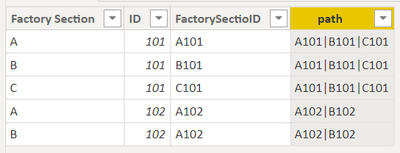cancel
Showing results for
Did you mean:Frequent Visitor

## IDs that change by location: Connecting IDs in a previous location to test data in a later location

I have an ID column where the ID of a part changes depending on which section of the factory it is in:

For example:

Say I have factory sections A, B, and C respectively in a process. The ID for a part will change like so: A101 --> B101 -->C101

If I have a test in section C that parts are failing for, how can I make sure that the defect test data is married to the previous IDs as well as the current one? If something in section A is the source, I need to be able to see which parts of section A had the most failures in section C. How can I do that when they are in the same column? (assume the actual IDs are more complicated)

1 ACCEPTED SOLUTIONSuper User

Hey @dotdotdashboard ,

not sure if I understood your requirement 100% correct, but nevertheless, I used the following DAX to create a new calculated column, this column groups the ids along the process you described (at least how I understood your explanation):

``````path =
var currentID = 'Table'[ID]
var factorySection = DATATABLE( "FactorySection", STRING , "Sequence" , INTEGER, {{"A" , 1} , {"B" , 2} , {"C" , 3}} )
var numberOfRows =
COUNTROWS(
FILTER(
'Table'
, 'Table'[ID] = currentID
)
)
return
CONCATENATEX(
FILTER(
factorySection
, [Sequence] <= numberOfRows
)
, [FactorySection] & [ID]
, "|"
, [Sequence]
, ASC
)``````

The DAX above assumes that there are columns containing the factory section and the ID. These columns can be created using PowerQuery by splitting a duplicate of the column into the factory segment and the id.

The result will look like this:You can use various functions to find the "SectionID" in the created string. Because I used the "|" the string can be considered a path, allowing for using functions like PATHCONTAINS and PATHLENGTH.

Hopefully, this provides some ideas on how to tackle your challenge.

Regards,

Tom

Did I answer your question? Mark my post as a solution, this will help others!

Proud to be a Super User!
I accept Kudos 😉
Hamburg, GermanySuper User

Hey @dotdotdashboard ,

not sure if I understood your requirement 100% correct, but nevertheless, I used the following DAX to create a new calculated column, this column groups the ids along the process you described (at least how I understood your explanation):

``````path =
var currentID = 'Table'[ID]
var factorySection = DATATABLE( "FactorySection", STRING , "Sequence" , INTEGER, {{"A" , 1} , {"B" , 2} , {"C" , 3}} )
var numberOfRows =
COUNTROWS(
FILTER(
'Table'
, 'Table'[ID] = currentID
)
)
return
CONCATENATEX(
FILTER(
factorySection
, [Sequence] <= numberOfRows
)
, [FactorySection] & [ID]
, "|"
, [Sequence]
, ASC
)``````

The DAX above assumes that there are columns containing the factory section and the ID. These columns can be created using PowerQuery by splitting a duplicate of the column into the factory segment and the id.

The result will look like this:You can use various functions to find the "SectionID" in the created string. Because I used the "|" the string can be considered a path, allowing for using functions like PATHCONTAINS and PATHLENGTH.

Hopefully, this provides some ideas on how to tackle your challenge.

Regards,

Tom

Did I answer your question? Mark my post as a solution, this will help others!

Proud to be a Super User!
I accept Kudos 😉
Hamburg, Germany